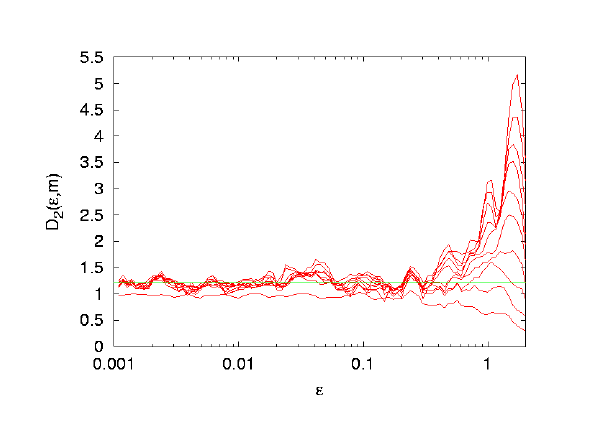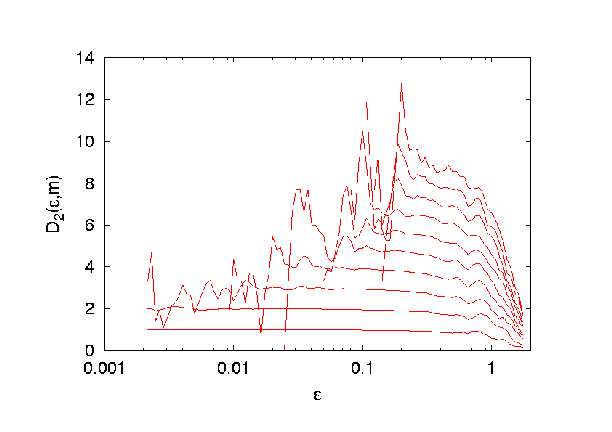# Description of the program: d2

This program estimates the correlation sum, the correlation dimension and the correlation entropy of a given, possibly multivariate, data set. It uses the box assisted search algorithm and is quite fast as long as one is not interested in large length scales. All length scales are computed simultaneously and the output is written every 2 min (real time, not cpu time). It is possible to set a maximum number of pairs. If this number is reached for a given length scale, the length scale will no longer be treated for the rest of the estimate.

Please consult the introduction paper for initial material on dimension estimation. If you are serious, you will need to study some of the literature cited there as well.

In extension to what is described there, the simultaneous use of multivariate data and temporal embedding is possible using d2. Thus one has to give two numbers in order to specify the embedding dimension. The first is the number of multivariate components, the second the number of lags in the temporal embedding. This is realized by giving two numbers to the option -M, seperated by a comma. For a standard scalar time series, set the first number to 1. If your multivariate data spans the whole phase space and no further temporal embedding is desired, set the second value to 1. In any case, the total embedding dimension in the sense of the embedding theorems is the product of the two numbers.

In order to be able to assess the convergence with increasing embedding dimension, results are reported for several such values. The inner loop steps through the number of components until the first argument of M is reached. The outer loop increases the number of time lags until the second argument of M is reached.

#### Usage of the -c and -M flags

Suppose, the option -M x,y has been specified. By default, the first x columns of a file are used. This behaviour can be modified by means of the -c flag. It takes a series of numbers separated by commas. The numbers represent the colomns. For instance -M 3,1 -c 3,5,7 means, use three components with no additional temporal embedding, reading columns 3, 5 and 7. It is not necessary to give the full number of components to the -c flag. If numbers are missing, the string is filled up starting with the smallest number, larger than the largest given. For instance, -M 3,1 -c 3 would result in reading columns 3, 4 and 5.

## Usage:

d2 [Options]

Everything not being a valid option will be interpreted as a potential datafile name. Given no datafile at all means read stdin. Also - means stdin

Possible options are:

Option Description Default
-l# number of data points to be used whole file
-x# number of lines to be ignored 0
-d# delay for the delay vectors 1
-M#,# # of components,maximal embedding dimension 1,10
-c# columns to be read 1,...,# of components
-t# theiler window 0
-R# maximal length scale (max data interval)
-r# minimal length scale (max data interval)/1000
-## number of epsilon values 100
-N# maximal number of pairs to be used
(0 means all possible pairs)
1000
-E use data that is normalized to [0,1] for all components not set (use natural units of the data)
-o[#] output file name (without extensions) 'datafile'[.c2][.d2][.h2][.stat]
(or if data were read from stdin: stdin[.c2][.d2][.h2][.stat])
-V# verbosity level
0: only panic messages
2: add input/output messages each time output file is opened
1
-h show these options none

## Description of the Output:

The files with the extensions c2, d2 and h2 contain for each embedding dimension and each length scale two columns:
first column: epsilon (in units chosen)
second column: the estimated quantity (correlation sum, dimension, entropy)
.
• extension .c2: This file contains the correlation sums for all treated length scales and embedding dimensions.
• extension .d2: This file contains the local slopes of the logarithm of the correlation sum, the correlation dimension.
• extension .h2: This file contains the correlation entropies.
• extension .stat: This file shows the current status of the estimate.
The output is written every two minutes (real time, not cpu time). So, you can see preliminary results even if the program is still running. Post-processing can be done in the following ways. Either of the output sequences can be smoothed by av-d2. Takens' estimator can be obtained from the correlation sum (extension .c2) using c2t and then plotted versus upper cut-off length. The Gaussian kernel correlation integral can be obtained from the standard correlation sum (extension .c2) using c2g.

## How to get D2 from the data

The file with the extension .d2 contains the skeleton to estimate the correlation dimension. As written above it contains the local slopes of the correlation sums for the different embedding dimension. To get an idea of the correlation dimension it is always a good idea to plot the file using a log scale for the x-axis.
The figures you get could look something like one of the following ones.The first figure clearly shows a plateau. This means for a wide range of length scales (x-axis) and for all embedding dimensions larger than a minimal one, the curves collapse and are flat. The value of the plateau gives an estimate for the dimension (1.2... in this case). The second figure does not give any dimension estimate, at all. All curves behave different and there is no common behaviour. From this figure one can not conclude any dimension for the system.
Typically one finds something in between the two extremes shown above.
View the C-source.